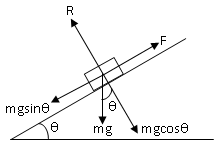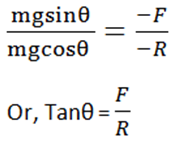## TyroCity

Physics XI Notes for Physics Notes

Posted on • Updated on

# Relation Between Angle Of Friction And Angle Of Repose

Angle of repose is defined as the minimum angle made by an inclined plane with the horizontal such that an object placed on the inclined surface just begins to slide.
Relation between Angle of Friction and Angle of Repose
Let us consider a body of mass ‘m’ resting on a plane.

Also, consider when the plane makes ‘θ’ angle with the horizontal, the body just begins to move.Let ‘R’ be the normal reaction of the body and ‘F’ be the frictional force.

Here,
mgsinθ = -F ——> (i)
mgcosθ = -R ——>(ii)

Dividing equation (i) by (ii)Or, Tanθ = µ, where ‘µ’ is the coefficient of friction
Or, Tanθ = Tanɑ (tanɑ = µ)

where ‘ɑ’ is the angle of friction

θ = ɑ
Angle of repose is equal to angle of friction.